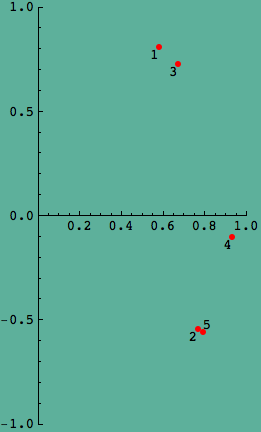## PCA / FA example 1: harman. discussion 2

recall that we had discovered that harman’s new F variables were uncorrelated and standardized: they had mean 0 and variance 1. he had implied, however, that the variances of the new variables would be the eigenvalues of the correlation matrix of Z.

he didn’t get that. can we?$Z = A F$

(where Z is the standardized data, A is a weighted eigenvector matrix, and F is the transformed, new, data), which implies$F^T = X \ A^{-T}$

we take$Z = P Y$,

(where P is an orthogonal eigenvector matrix, and Y is the transformed, new, data), i.e.$Z^T = Y^T \ P^T$

hence$Y^T = Z^T \ P^{-T} = X P$.

(in words, we fix his messy$A^{-T}$ by using an orthogonal eigenvector matrix P, for which$P^{-T}= P$.)
Read the rest of this entry »

## biblio is now a page…

well, it just seemed better to make it a page. and it seemed worth doing as i added a few more books. after it gets bigger, i’ll be able to create subpages for specialized bibliographies. i’ve been too busy lately. adding a few books was something i could do in the evenings.

i’ve had a friend visiting for a while, and i had better things to do than math. oh, math is good, but friends are better. in moderation.

## PCA / FA Example 1: Harman. discussion 1.

harman gave us two outputs as a result of his analysis: a table and a picture.

let’s consider the picture first. it clearly shows z2 and z5 similar, z1 and z3 similar, and z4 roughly in the middle between them.i don’t know about you, but this surprised me. should it have? well, let’s take a look at the correlation matrix, from which we got our results. i’m going to round it off just a little bit, so i can refer to 3-digit numbers instead of 5.
Read the rest of this entry »

## PCA / FA Example 1: Harman. what he did.

Less is more. And more is huge. It is easy for me to end up with huge posts to put out here, but I’d rather go with smaller.

Let’s get started with PCA / FA, principal components analysis and factor analysis.

In case it matters, I am using Mathematica to do these computations.

Here is an example, the first of several. This comes from Harman’s “factor analysis”. In order to emphasize the distinction between PCA and FA, he has one example of principal component analysis, and this is it.

Let me tell you up front what he did:

• Get some data;
• Compute its correlation matrix;
• Find the eigenstructure of the correlation matrix;
• Weight each eigenvector by the square root of its eigenvalue;
• Tabulate the results;
• Plot the original variables in the space of the two largest principal components.

I also need to say that his conceptual model is written

Z = A F,

And from the dimensions of the matrices it is clear that

A is square, k by k

Z and F are the same shape, with k rows.

We infer from its size that A will be derived from the eigenvector matrix, and that Z is derived from the given data matrix. From the shapes, we conclude that Z has observations in columns, rather than in rows. (If you’re used to econometrics or regression, you expect the transpose, observations in rows.)

But this is a fine thing, because we recognize that Z = A F is a change-of-basis equation for corresponding columns of Z and F; A is a transition matrix mapping new components (any one column of F) to old components (a column of Z).

## the poincare’ conjecture & other stuff

Ok, the holidays are behind me. One friend called me to make sure I was ok, because I had made no blog entries in two weeks. Well, my Xmas letter took a little longer than usual this year; and there was the return to work. I think that trying to write about mathematics had affected my letter-writing style, and I had to work at recovering it. For the Xmas letter, one of my guiding principles is to not take myself too seriously.

For this blog, however, I worry about the line between seeming all-too-human and seeming off-the-wall. What comes across as human in a personal letter may seem flaky in mathematics. Maybe I’ll just have to be myself and leave it for you to judge.

Donal O’Shea’s “the Poincare’ conjecture” arrived from Amazon Wednesday. I read chapters 1 & 2 before getting caught up in routine, then read the rest of the book last night (Friday).